﻿ 改进区块遗传算法解决分布式车间调度问题
«上一篇文章快速检索 高级检索

 智能系统学报2021, Vol. 16Issue (2): 303-312  DOI: 10.11992/tis.2019060350

### 引用本文PEI Xiaobing, SUN Zhiwei. Solving distributed-shop scheduling problems based on modified genetic algorithm[J]. CAAI Transactions on Intelligent Systems, 2021, 16(2): 303-312. DOI: 10.11992/tis.201906035.### 文章历史

Solving distributed-shop scheduling problems based on modified genetic algorithm
PEI Xiaobing , SUN ZhiweiSchool of Management, Tianjin University of Technology, Tianjin 300384, China
Abstract: To solve distributed-job-shop scheduling problems, in this paper, we propose a modified block-genetic algorithm (MBGA). First, we initialize the solutions by combining NEH and random assignments. Then, by statistical analysis, we select an elite chromosome to establish a job-factory distribution matrix and job-machine sorting matrix to mine the block’s closely linked gene chain. Block-based artificial chromosomes are constructed and recombined to improve the quality and diversity of the solutions. The experimental results show that the performance of the proposed MBGA algorithm is superior to that of other well-known algorithms with respect to its stability and accuracy.
Key words: block    synergistic effect    artificial chromosomes    distributed job shop scheduling problem    genetic algorithm    gene recombination    probability matrix    combinatorial optimization

1 数学模型的阐述

 ${\rm{Minimize}}\;{{{C}}_{{\rm{max}}}}$ (1)

Subject to:

 $\sum_{r = 1}^f {{Y_{j,r}}} = 1,{\rm{ }}{\forall _{{j}}}$ (2)

 ${C_{j,i}} \geqslant {p_{j,i}} , {\forall _j}_{,i}$ (3)

 ${C_{j,i}} \geqslant {C_{j,l}} + {p_{j,i}}{\rm{ , }}{\forall _{j,i,l \ne i\left| {{a_{j,i,l}} = 1} \right.}}$ (4)

 ${C_{j,i}} \geqslant {C_{k,i}} + {p_{j,i}} - M(3 - {X_{k,j,i}} - {Y_{j,r}} - {Y_{k,r}}),{\forall _{{{r,i,k}} < n,j > k}}$ (5)
 ${C_{k,i}} \geqslant {C_{j,i}} + {p_{k,i}} - M(2 + {X_{k,j,i}} - {Y_{j,r}} - {Y_{k,r}}),{\forall _{{{r,i,k}} < n,j > k}}$ (6)

 ${C_{\max }} \geqslant {C_{j,i}}{\rm{ }},{\forall _{{{j,i}}}}$ (7)

 ${C_{j,i}} \geqslant 0 {\forall _{j,i}}$ (8)
 ${Y_{j,i}} \in \{ 0,1\} {\rm{ , }}{\forall _{{{j,i}}}}$ (9)
 ${X_{k,j,i}} \in \{ 0,1\} ,{\forall _{j < n,k > j,i}}$ (10)

2 改进区块遗传算法

1)生成初始解。为保证初始解的质量和多样性，本文运用NEH和随机性两种方式，按照最早完工的原则初始化种群。

2)计算适应度并挑选精英染色体。计算每个染色体的适应值，并使适应度按从小到大的顺序进行排序，挑选出前30%的染色体作为精英染色体。

3)更新概率矩阵。分别建立工件−车间分配矩阵和工件−机器排序矩阵，并随着迭代过程不断地更新概率矩阵中的信息。

4)组合区块。根据概率矩阵中的信息，组合区块。

5)构建人工染色体。根据区块库和概率矩阵中的信息，运用轮盘赌的方式构建人工染色体。

6)对分布式车间调度问题的解序列进行重组。将父代的染色体以某一车间中工件加工的解序列片段为单位进行重组。

7)筛选优精英染色体。将从重组后的染色体与原父代的染色体进行融合，筛选出新的精英染色体作为下一次迭代的父代。

MBGA算法流程图(见图1)中， $\Delta R$ 为概率模型迭代计数器， ${R_{{\rm{the}}}}$ 为概率模型迭代的门槛值， $\Delta A$ ${A_{{\rm{the}}}}$ 分别为构成人工染色体的计数器和门槛值。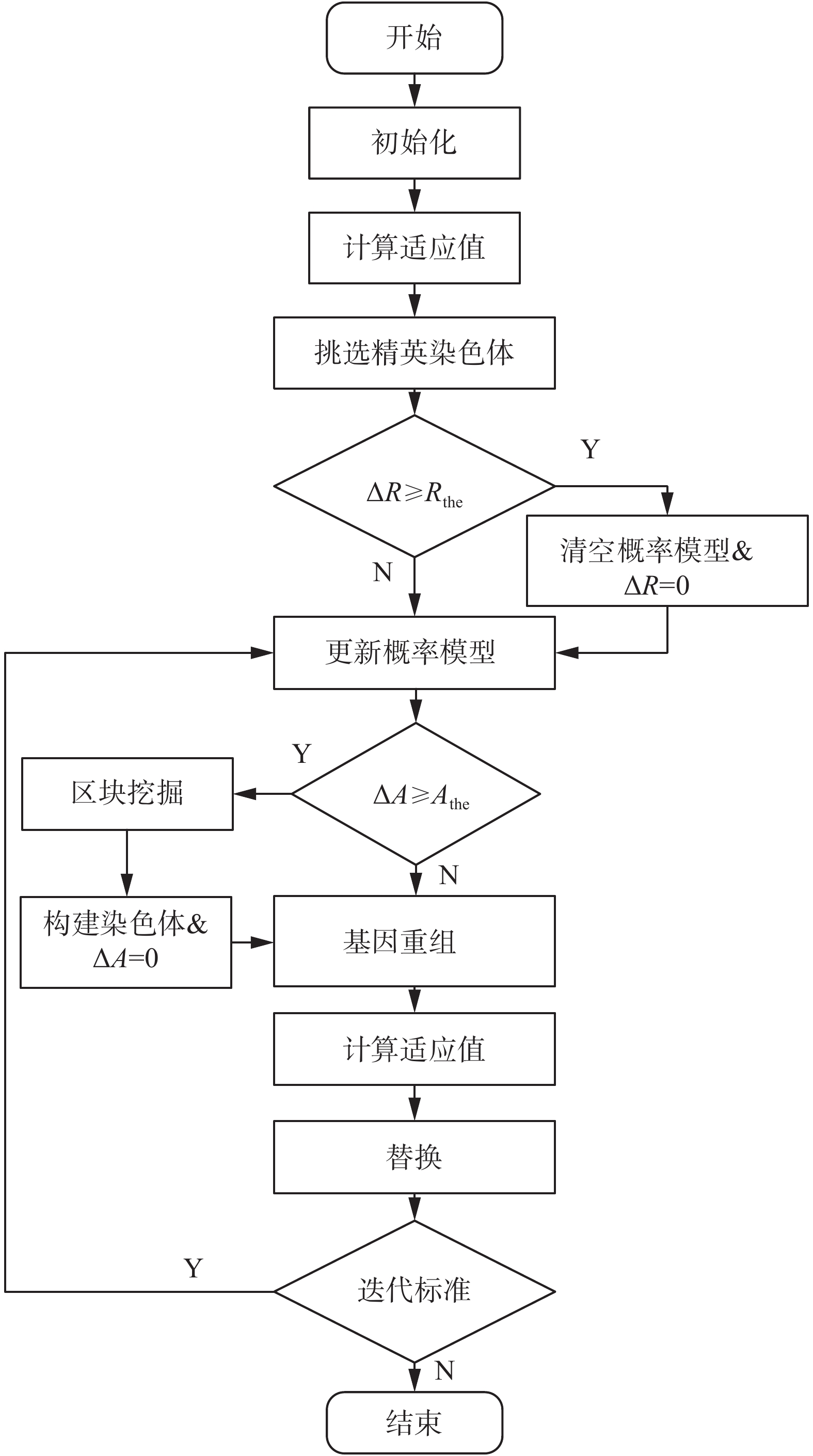Download: 图 1 MBGA流程 Fig. 1 Flow chart of MBGA
2.1 生成初始解

 ${{\rm{workload}}_{(j,i)}} = \bigg(\sum\limits_{k \in {R_{j,i}}} {{p_{j,k}}} \bigg) + {p_{j,i}},{\rm{ }}{\forall _{i,j}}$ (11)表 1 分布式工作车间中工件的加工时间和路径 Tab.1 Processing time and path in distributed job shop表 2 优先排序权 Tab.2 Job priority
2.2 构建概率矩阵模型

 $\begin{array}{l} X_{ir}^k = \left\{ \begin{array}{l} \begin{array}{*{20}{c}} \!\!\!\!\!\!\!\!1,\quad{{\text{如果工件}}i{\rm{ }}{\text{在工厂}}r} \end{array}\\ \begin{array}{*{20}{c}} \!\!\!\!\!\!\!\!0,\quad{{\text{其他}}} \end{array} \end{array} \right.\\ i = 1,2, \cdots, n;\;{{ r}} = 1,2, \cdots, f;{\rm{ }}k = 1,2, \cdots, s \end{array}$ (12)
 $\begin{array}{l} {T_{ir}}(t) = {T_{ir}}(t - 1) + \displaystyle \sum\limits_{k = 1}^m {X_{ir}^k} ,{\rm{ }}i = 1,2, \cdots, n;\\ r = {\rm{1}},2, \cdots ,f;{\rm{ }}t = 1,2, \cdots, G;{\rm{ }}k = 1,2, \cdots, s \end{array}$ (13)

 $\begin{array}{l} X_{ir}^k = \left\{ \begin{array}{l} \begin{array}{*{20}{c}} \!\!\!\!\!\!\!\!1,\quad{{\text{如果工件}}i{\rm{ }}{\text{在机器}}j} \end{array}\\ \begin{array}{*{20}{c}} \!\!\!\!\!\!\!\!0,\quad{{\text{其他}}} \end{array} \end{array} \right.\\ i = 1,2, \cdots, n;{\rm{ }}j = 1,2,\cdots, n;{\rm{ }}k = 1,2, \cdots, m \end{array}$ (14)
 $\begin{array}{l} {T_{ij}}(t) = {T_{ij}}(t - 1) + \displaystyle \sum\limits_{k = 1}^m {X_{ij}^k} {\rm{ }},i = 1,2, \cdots, n;\\ j = 1 ,2,\cdots, n;{\rm{ }}t = 1,2, \cdots, G;{\rm{ }}k = 1,2, \cdots, s \end{array}$ (15)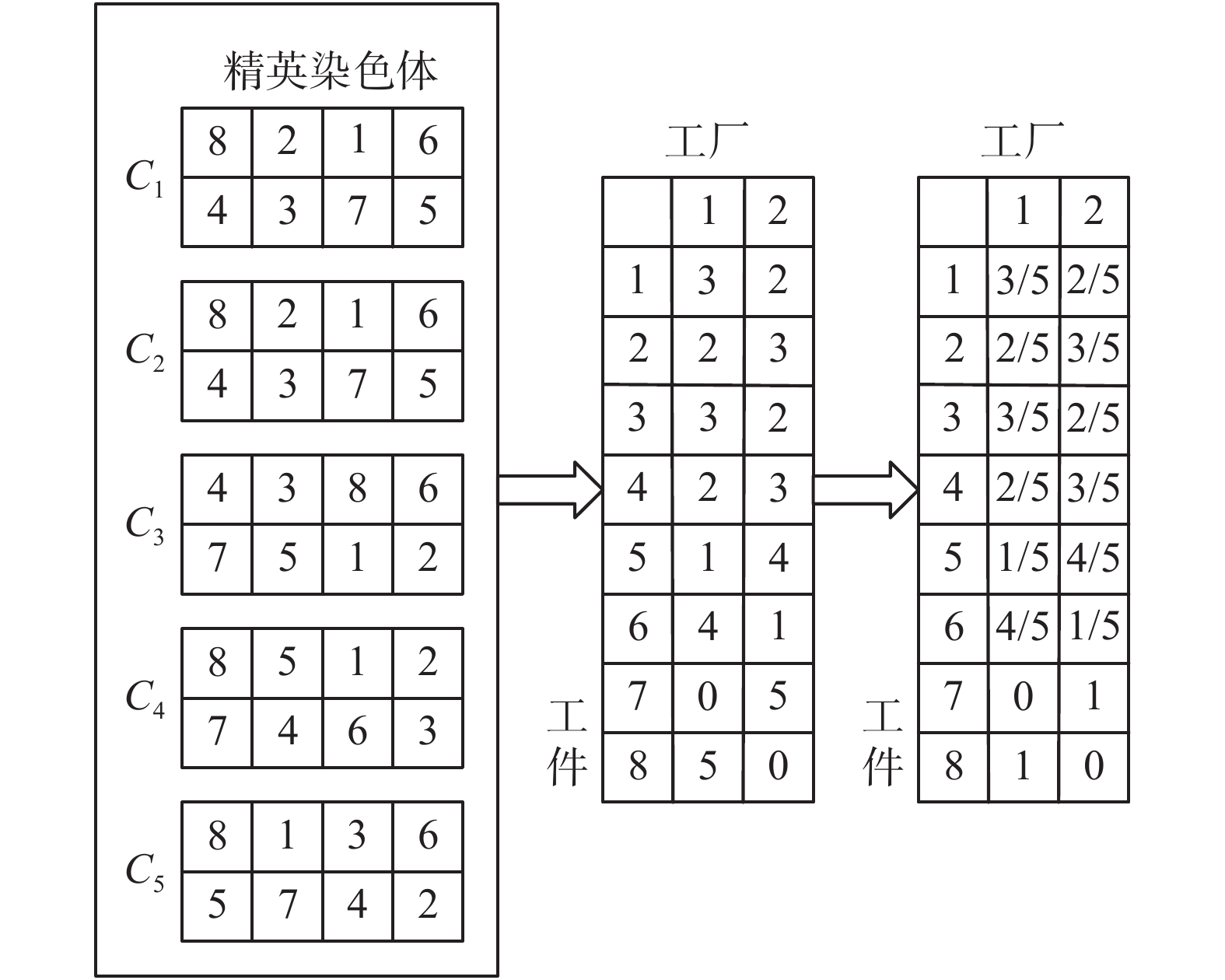Download: 图 2 工件−车间分配概率矩阵示意 Fig. 2 Schematic diagram of job-shop assignment probability matrix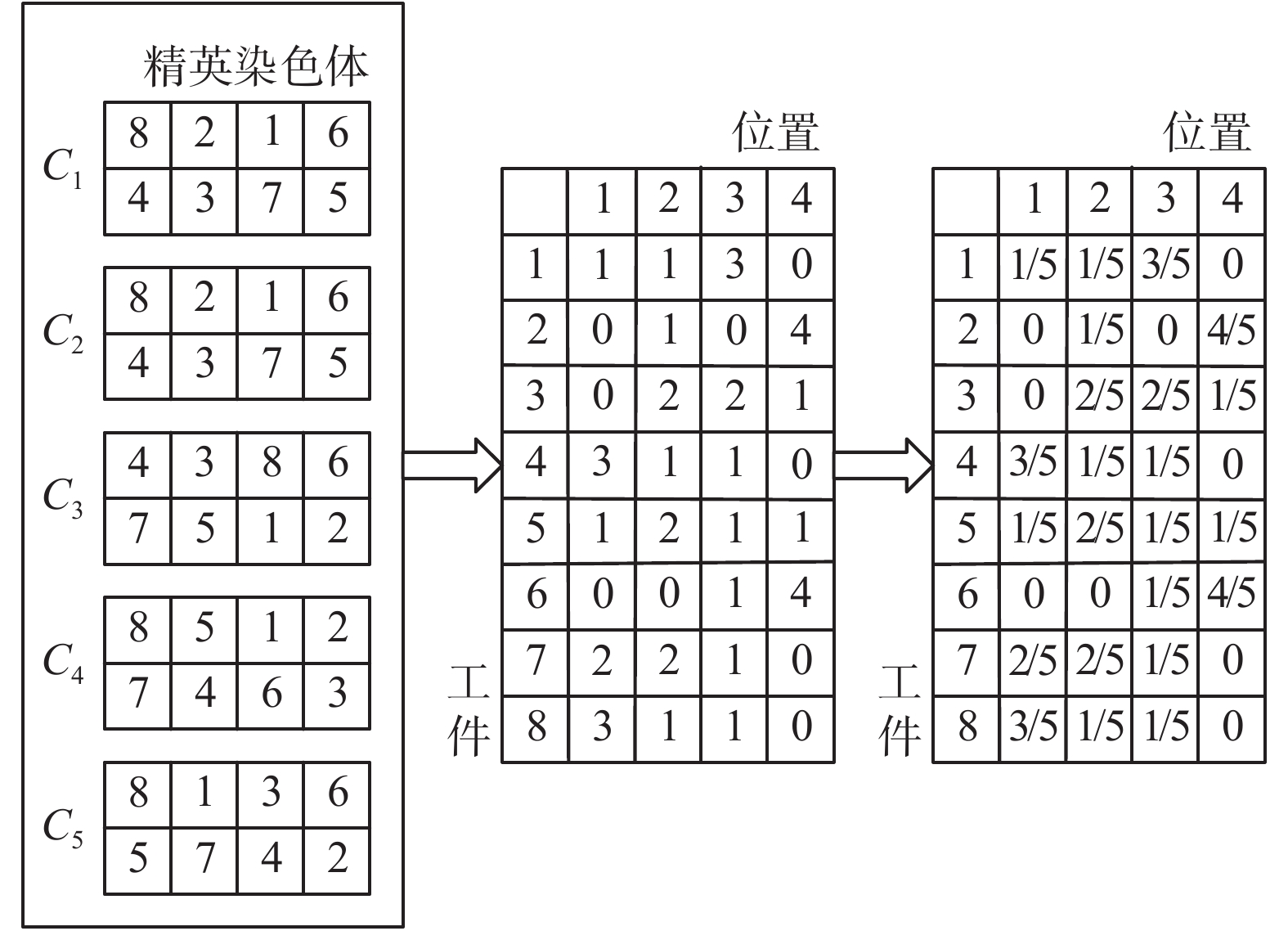Download: 图 3 工件−位置排序矩阵示意 Fig. 3 Schematic diagram of job-position sorting matrix
2.3 区块挖掘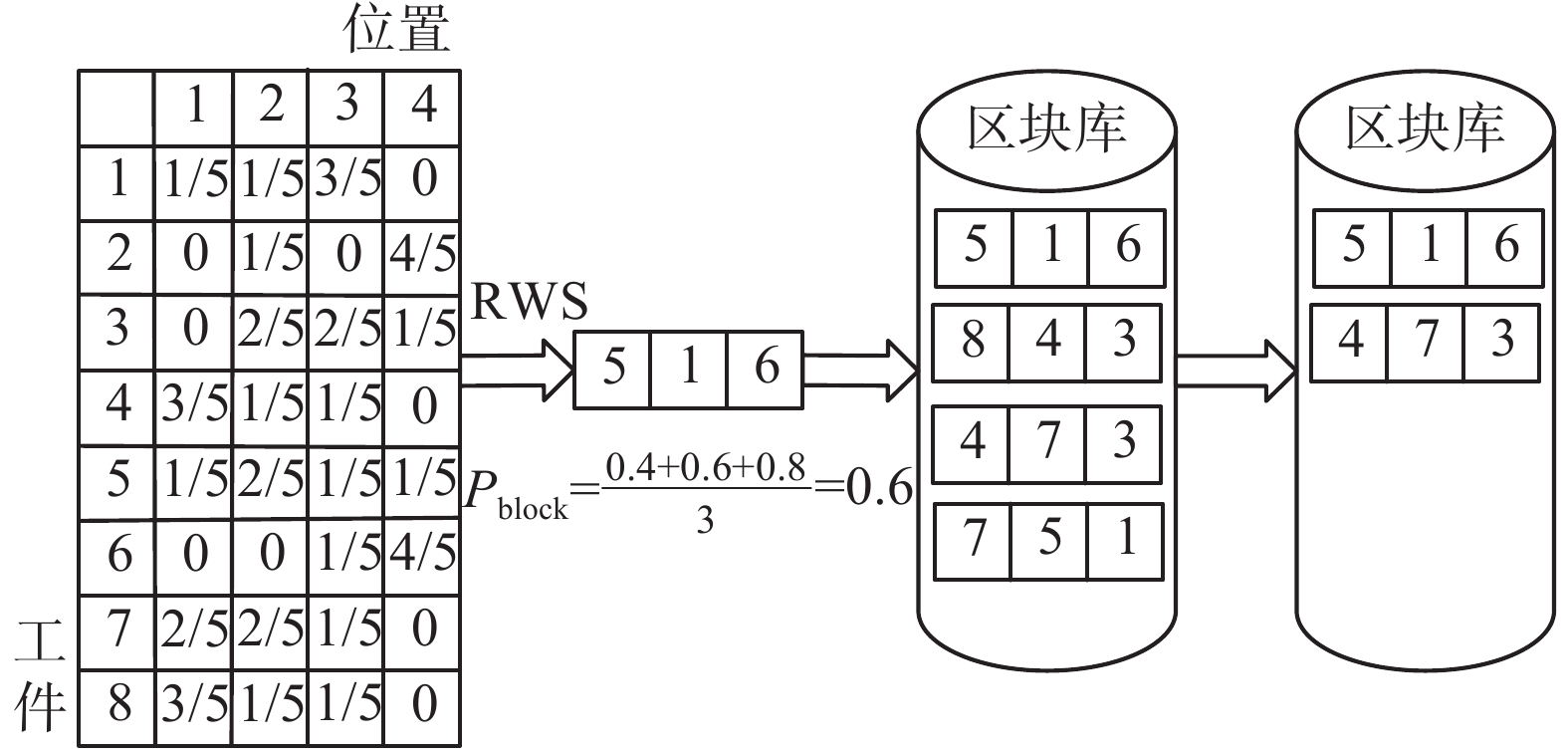Download: 图 4 工件−车间分配区块挖掘方式 Fig. 4 Job-shop assignment block mining method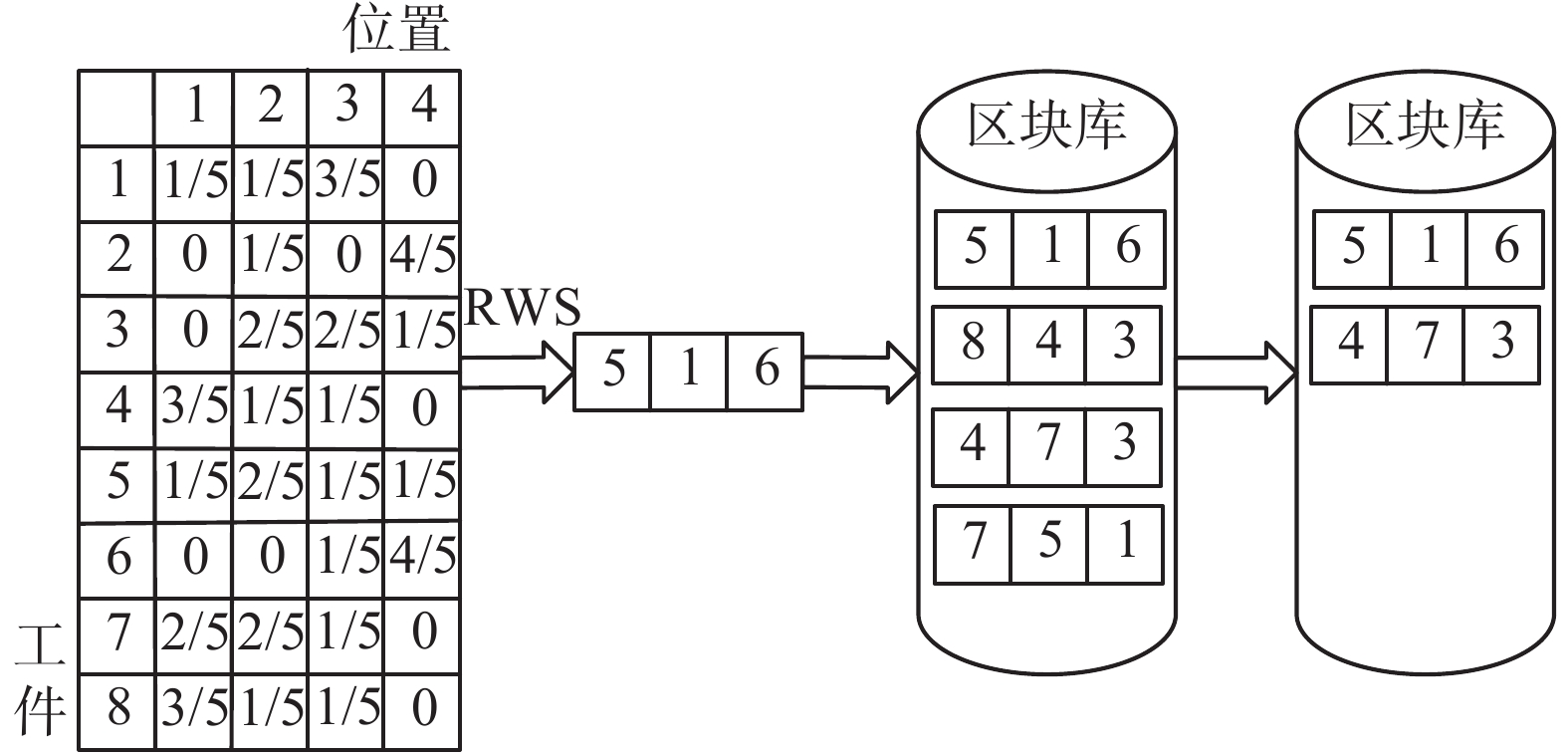Download: 图 5 工件−位置排序区块挖掘方式 Fig. 5 Job-location sorting block Mining method

 $P_{{B^{(n)}}}^{{\rm{avg}}} = {L^{ - 1}}\sum\limits_{i = 1}^L {P_{B_i^{(n)}}^{(p)}}$

2.4 构建人工染色体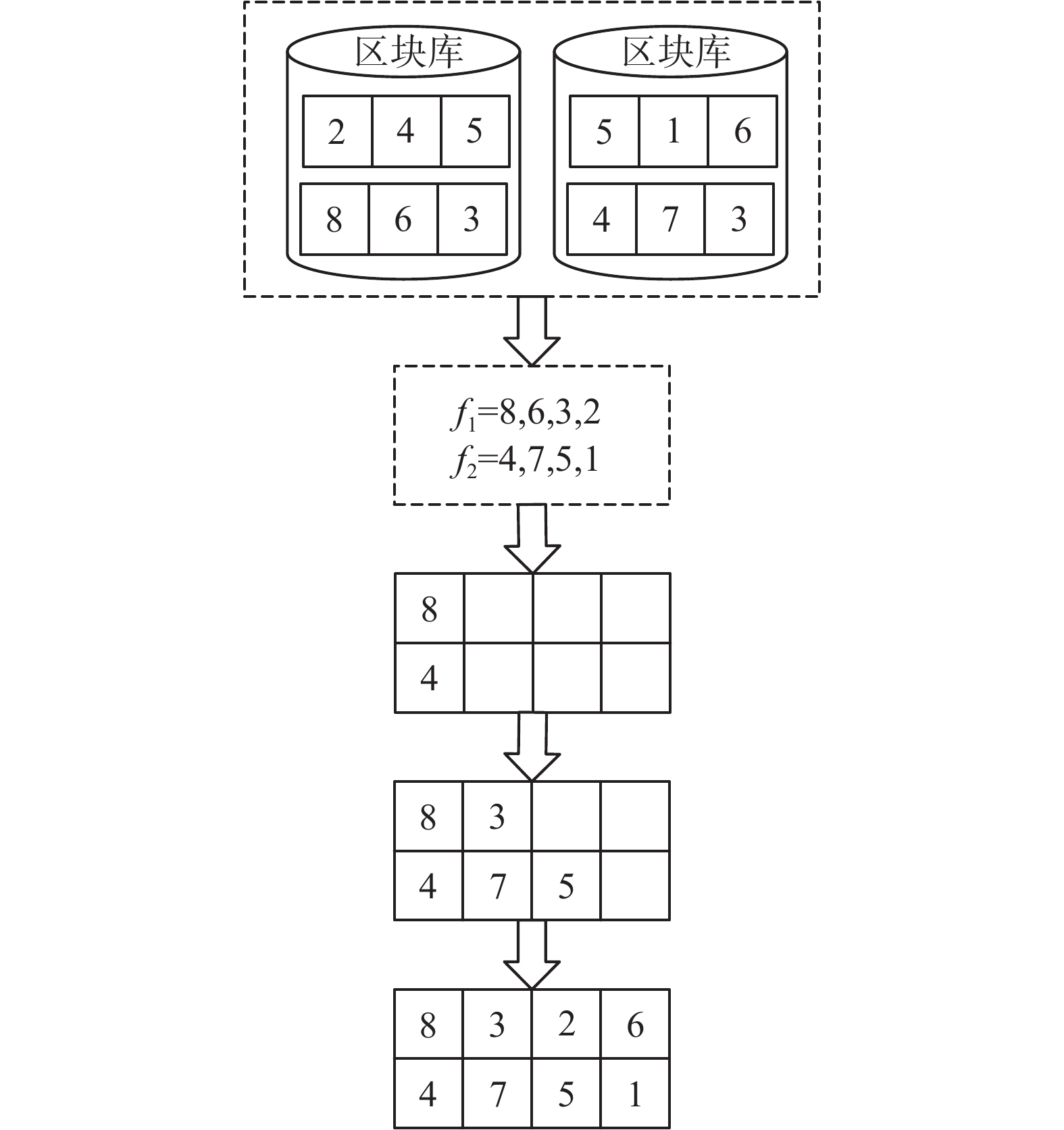Download: 图 6 第1种组合机制 Fig. 6 The first combination mechanism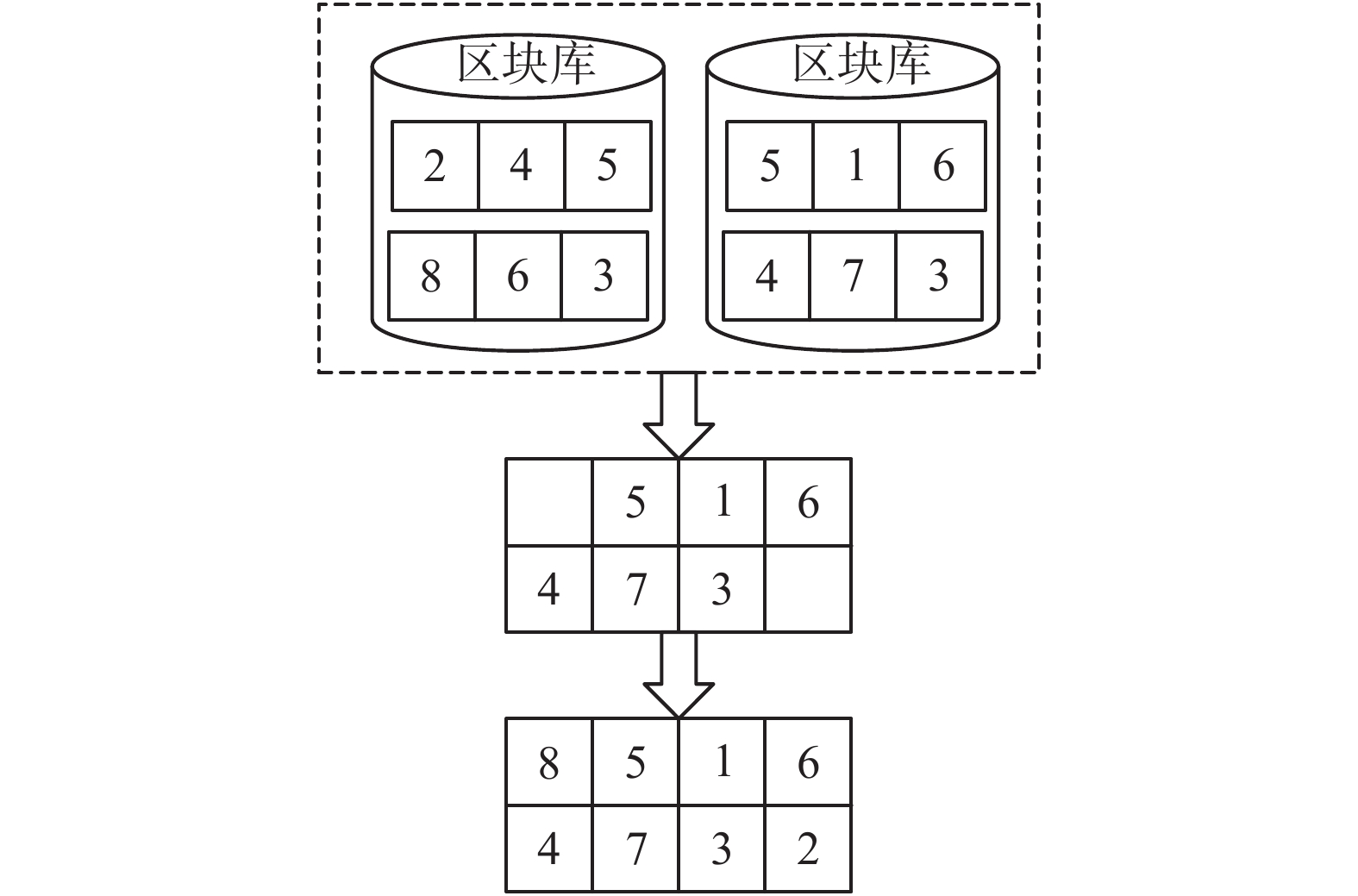Download: 图 7 第2种组合机制 Fig. 7 The second combination mechanism
2.5 基因重组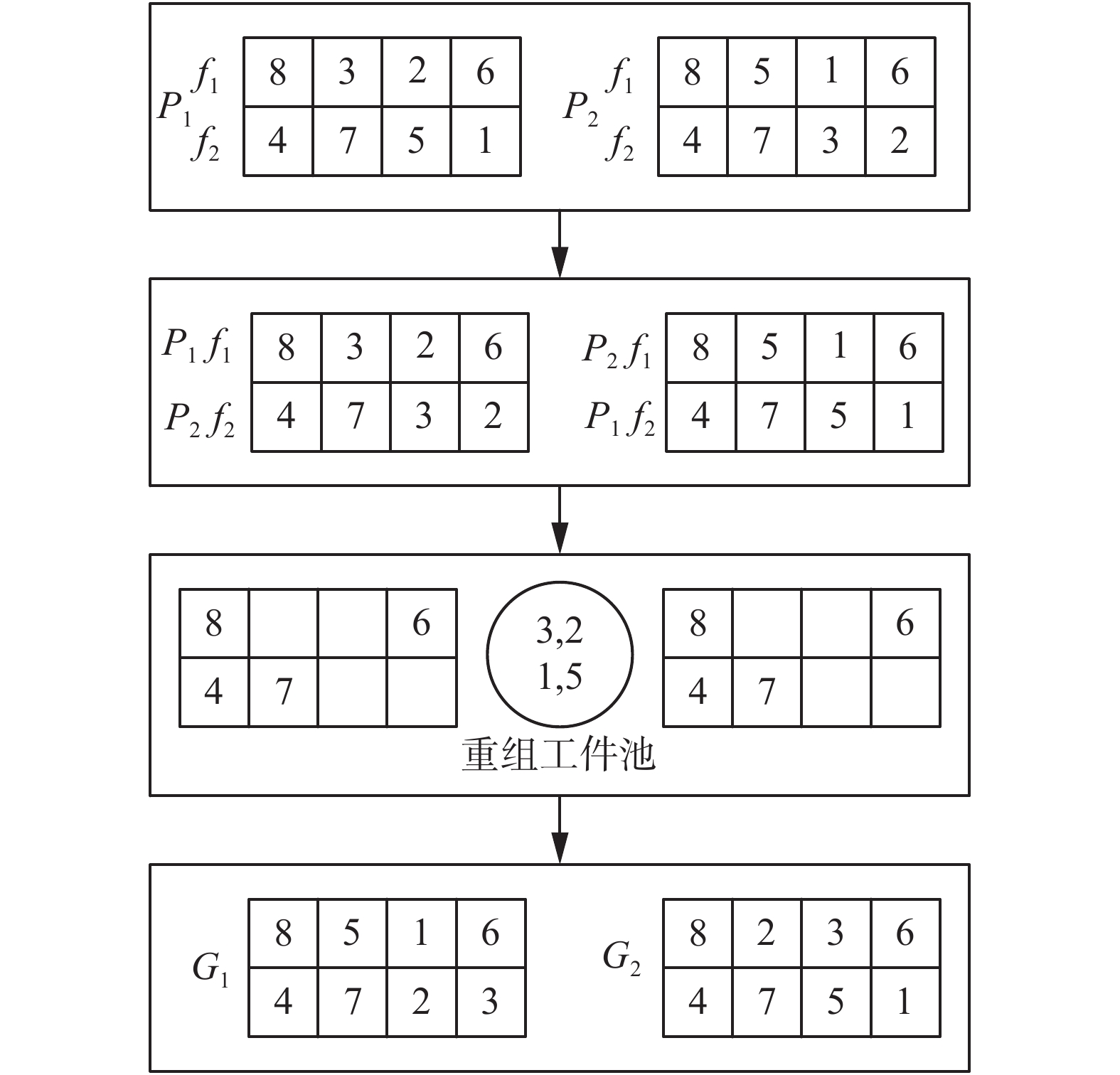Download: 图 8 基因交叉 Fig. 8 Gene crossing
3 计算结果与分析

 ${\rm{RPD}} = \frac{{{\rm{A}}\lg - {\rm{Min}}}}{\rm{Min}} \times 100$表 3 实例测试结果 Tab.3 Example test results表 4 不同算法的RPD Tab.4 RPD of different algorithms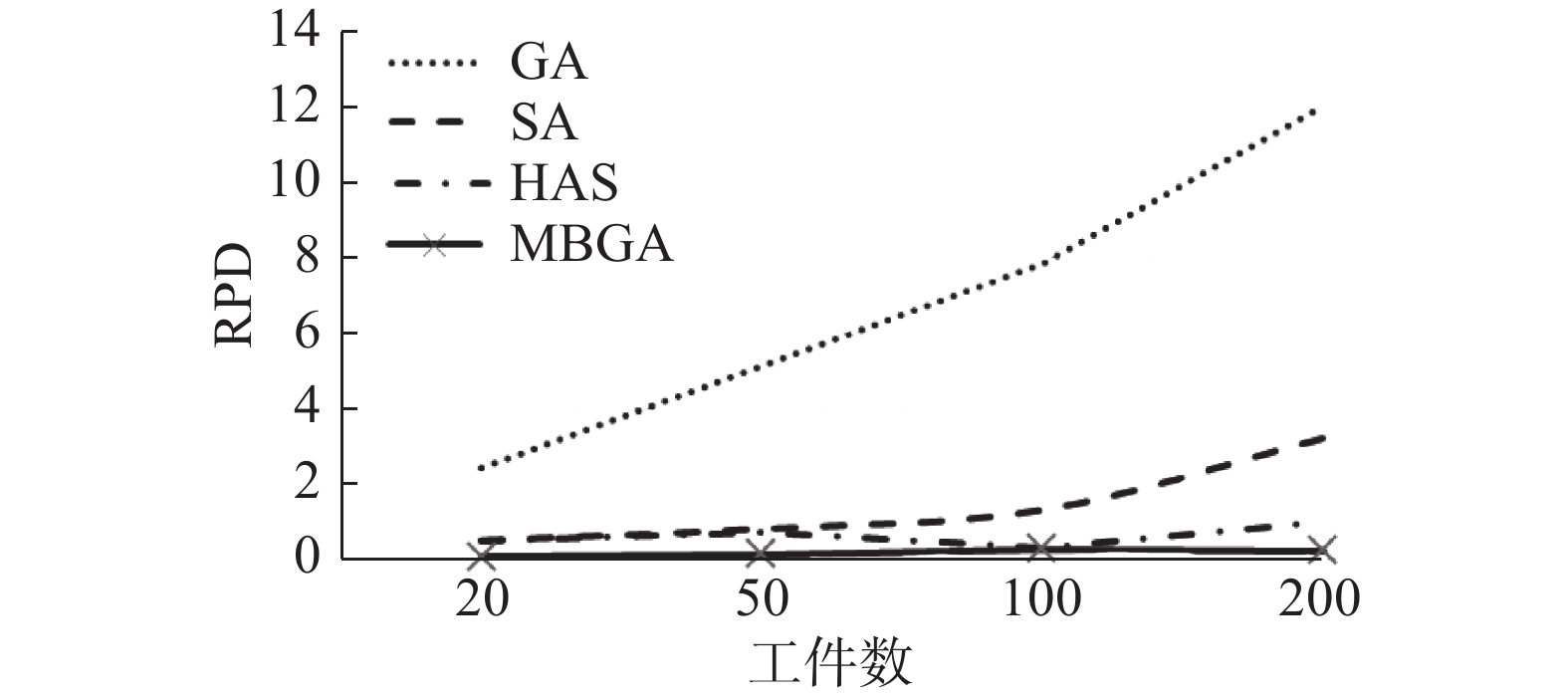Download: 图 9 平均RPD与工件数的关系 Fig. 9 Relationship between average RPD and number of jobs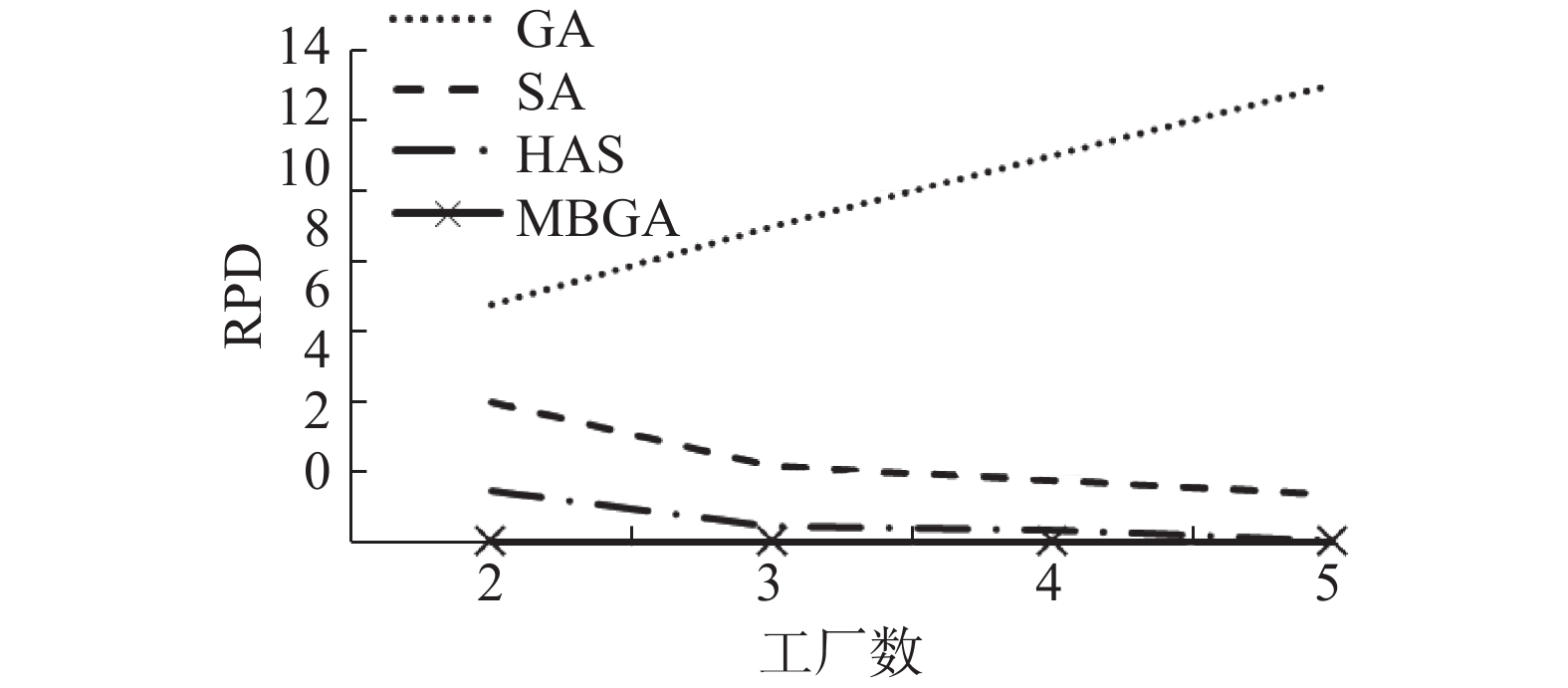Download: 图 10 平均RPD与车间数的关系 Fig. 10 Relationship between average RPD and the number of jobs
4 结束语

MBGA有效地解决了分布式生产车间调度问题，未来还可以尝试深入研究，如改变假设条件，当每个车间的工件的加工能力存在差异时，求解最优的调度方案。

  JIA H Z, FUH J Y H, NEE A Y C, et al. Web-based multi-functional scheduling system for a distributed manufacturing environment[J]. Concurrent engineering, 2002, 10(1): 27-39. DOI:10.1177/1063293X02010001054 (0)  谢志强, 周含笑, 桂忠艳, 等. 基于拟关键路径的二车间综合调度算法[J]. 计算机科学, 2013, 40(4): 193-198. XIE Zhiqiang, ZHOU Hanxiao, GUI Zhongyan, et al. Integrated scheduling algorithm of two workshops based on ACPM[J]. Computer science, 2013, 40(4): 193-198. DOI:10.3969/j.issn.1002-137X.2013.04.042 (0)  BARGAOUI H, DRISS O B, GHÉDIRA K. Minimizing makespan in multi-factory flow shop problem using a chemical reaction metaheuristic[C]//Proceedings of 2016 IEEE Congress on Evolutionary Computation. Vancouver, Canada, 2016. (0)  谢志强, 郭禾, 苏文秀, 等. 存在多工序同时结束的多车间逆序综合调度算法[J]. 吉林大学学报(工学版), 2018, 48(2): 578-587. XIE Zhiqiang, GUO He, SU Wenxiu, et al. Reversal sequence integrated scheduling algorithm of multiple workshop with multi-procedures ended together[J]. Journal of Jilin University (Engineering and Technology Edition), 2018, 48(2): 578-587. (0)  王凌, 邓瑾, 王圣尧. 分布式车间调度优化算法研究综述[J]. 控制与决策, 2016, 31(1): 1-11. WANG Ling, DENG Jin, WANG Shengyao. Survey on optimization algorithms for distributed shop scheduling[J]. Control and decision, 2016, 31(1): 1-11. (0)  AZAB A, NADERI B. Greedy heuristics for distributed job shop problems[J]. Procedia CIRP, 2014, 20: 7-12. DOI:10.1016/j.procir.2014.05.025 (0)  ZHANG Rui, SONG Shiji, WU Cheng. A dispatching rule-based hybrid genetic algorithm focusing on non-delay schedules for the job shop scheduling problem[J]. The international journal of advanced manufacturing technology, 2013, 67(1/2/3/4): 5-17. DOI:10.1007/s00170-013-4751-1 (0)  GAREY M R, JOHNSON D S, SETHI R. The complexity of flowshop and jobshop scheduling[J]. Mathematics of operations research, 1976, 1(2): 117-129. DOI:10.1287/moor.1.2.117 (0)  WAGNER H M. An integer linear-programming model for machine scheduling[J]. Naval research logistics quarterly, 1959, 6(2): 131-140. DOI:10.1002/nav.3800060205 (0)  JIA H Z, NEE A Y C, FUH J Y H, et al. A modified genetic algorithm for distributed scheduling problems[J]. Journal of intelligent manufacturing, 2003, 14(3/4): 351-362. DOI:10.1023/A:1024653810491 (0)  王和敏, 谷淼, 魏志谦, 等. 基于混合遗传算法的Job Shop问题研究与实现[J]. 新技术新工艺, 2017(8): 69-73. WANG Hemin, GU Miao, WEI Zhiqian, et al. The Research and implement of Job Shop problem based on hybrid genetic algorithm[J]. New technology & new process, 2017(8): 69-73. (0)  NADERI B, AZAB A. Modeling and heuristics for scheduling of distributed job shops[J]. Expert systems with applications, 2014, 41(17): 7754-7763. DOI:10.1016/j.eswa.2014.06.023 (0)  CHAOUCH I, DRISS O B, GHEDIRA K. A modified ant colony optimization algorithm for the distributed job shop scheduling problem[J]. Procedia computer science, 2017, 112: 296-305. DOI:10.1016/j.procs.2017.08.267 (0)  NADERI B, AZAB A. An improved model and novel simulated annealing for distributed job shop problems[J]. The international journal of advanced manufacturing technology, 2015, 81(1/2/3/4): 693-703. DOI:10.1007/s00170-015-7080-8 (0)  杨敬松, 夏秀峰, 崔广才. 混合遗传算法在分布式车间作业调度中的应用[J]. 计算机工程与应用, 2005, 41(19): 213-215, 225. YANG Jingsong, XIA Xiufeng, CUI Guangcai. The Distribution method of planning and scheduling based on a hybrid genetic algorithm[J]. Computer engineering and applications, 2005, 41(19): 213-215, 225. DOI:10.3321/j.issn:1002-8331.2005.19.062 (0)  高雪瑶, 谭涛, 张春祥. 遗传退火算法的模型相似性计算方法[J]. 哈尔滨工程大学学报, 2020, 41(7): 1073-1079. GAO Xueyao, TAN Tao, ZHANG Chunxiang. Method of calculating model similarity based on genetic annealing algorithm[J]. Journal of Harbin Engineering University, 2020, 41(7): 1073-1079. (0)  CHANG P C, HUANG W H, WU J L, et al. A block mining and re-combination enhanced genetic algorithm for the permutation flowshop scheduling problem[J]. International journal of production economics, 2013, 141(1): 45-55. DOI:10.1016/j.ijpe.2012.06.007 (0)  CHANG P C, CHEN Menghui. A block based estimation of distribution algorithm using bivariate model for scheduling problems[J]. Soft computing, 2014, 18(6): 1177-1188. DOI:10.1007/s00500-013-1136-1 (0)  裴小兵, 于秀燕. 改进猫群算法求解置换流水车间调度问题[J]. 智能系统学报, 2019, 14(4): 769-778. PEI Xiaobing, YU Xiuyan. Improved cat swarm optimization for permutation flow shop scheduling problem[J]. CAAI transactions on intelligent systems, 2019, 14(4): 769-778. (0)  张敏, 汪洋, 方侃. 基于改进区块进化算法求解置换流水车间问题[J]. 计算机集成制造系统, 2018, 24(5): 1207-1216. ZHANG Min, WANG Yang, FANG Kan. Improved block-based evolutionary algorithm for solving permutation flowshop scheduling problem[J]. Computer integrated manufacturing system, 2018, 24(5): 1207-1216. (0)  裴小兵, 张春花. 应用改进区块遗传算法求解置换流水车间调度问题[J]. 智能系统学报, 2019, 14(3): 541-550. PEI Xiaobing, ZHANG Chunhua. An improved puzzle-based genetic algorithm for solving permutation flow-shop scheduling problems[J]. CAAI transactions on intelligent systems, 2019, 14(3): 541-550. (0)  CHAOUCH I, DRISS O B, GHEDIRA K. A survey of optimization techniques for distributed job shop scheduling problems in multi-factories[C]//Proceedings of the 6th Computer Science On-line Conference. Cham, Germany, 2017. (0)